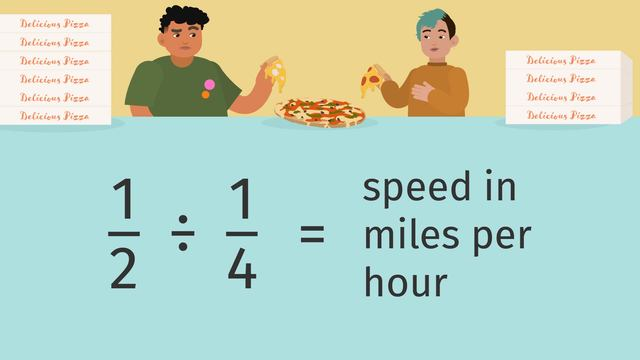# Unit Rates with FractionsRating

Be the first to give a rating!
The authorsTeam Digital
Unit Rates with Fractions
CCSS.MATH.CONTENT.7.RP.A.1

## Basics on the topicUnit Rates with Fractions

Unit Rates with Fractions - Given a ratio you can find the unit rate

### TranscriptUnit Rates with Fractions

Kai and Luis are interviewing for a pizza delivery job at Mario’s Pizza Palace. The person that can deliver at the fastest rate will be given the position, so let's compare the, Unit Rates with Fractions. To compare the delivery speeds, you need to find the unit rate this shows the quantity of a unit per one quantity of another unit. To find the unit rate, you set up a ratio and then divide the numerator by the denominator. It looks like the first orders are in, so Kai will get ready for the first delivery. Kai covers one-half of a mile in one-fourth of an hour, delivering pizzas to hungry customers. To find the unit rate for Kai’s delivery speed, you need to first set up a ratio, and then divide the miles by the hours. It's called a complex fraction when there is a fraction in the numerator or the denominator, or both. A complex fraction can simply be rewritten horizontally, to show that this is a division problem. Let's calculate Kai’s unit rate by dividing one-half by one-fourth, which will tell you what their speed is in miles per hour. Remember, when dividing fractions we multiply the first fraction by the reciprocal of the second fraction. Now multiply the numerators and denominators straight across, which equals four halves. Four halves simplifies to two. Kai delivered pizzas at a rate of two miles per hour. Now it's Luis's turn to deliver! Luis delivers the ordered pizzas flawlessly and covers one-third of a mile in one-sixth of an hour. Again, to find the unit rate, you divide the miles by the hours. You rewrite it like this and then change it to a multiplication problem. One-third multiplied by six is six-thirds which will simplify to two. Kai and Luis both had the same delivery speed of two miles per hour, resulting in a tie! Time for a rematch! Kai is handed the next set of orders, determined to beat their earlier delivery speed. Kai covers one mile in one-fourth of an hour. How do we find the unit rate? We divide one by one-fourth, and rewrite it as one multiplied by four. What is the unit rate? Four miles per hour, which is a new record for Kai! Luis’ delivery route has a whopping four miles on it, and he completes the order in one-fifth of an hour. Pause here and to calculate Luis' delivery speed to see if he, by some miracle, beat Kai’s record. Four divided by one-fifth is rewritten as four multiplied by five, which is twenty. Luis made his deliveries in an astonishing twenty miles per hour! Before we see who gets the job, let's summarize! Find the unit rate by changing the division of fractions to multiplying by the reciprocal. Finding the unit rate is useful when comparing different rates, such as miles and hours. Luis sure knows the meaning of working smarter, not harder.